6 Jul, 2015

# How to Estimate Compressor Efficiency

In the November 2011 tip of the month (TOTM) we presented the compressor calculations of a case study. We compared the rigorous method results with the values from the shortcut methods. The rigorous method was based on an equation of state like the Soave-Redlich-Kwong (SRK) for calculating the required enthalpies and entropies. The enthalpies and entropies are used to determine the power requirement and the discharge temperatures. The results indicated that the accuracy of the shortcut method is sensitive to the value of ideal gas state heat capacity ratio, k.

From a calculation viewpoint alone, the power calculation is particularly sensitive to the specification of mass flow rate, suction temperature and pressure, and discharge temperature and pressure. A compressor is going to operate under varying values of the variables affecting its performance. Thus the most difficult part of a compressor calculation is specification of a reasonable range for each variable and not the calculation itself. Reference  emphasizes that using a single value for each variable is not the correct way to evaluate a compression system.

Normally, the thermodynamic calculations are performed for an ideal (reversible) process. The results of a reversible process are then adapted to the real world through the use of a thermodynamic efficiency. In the compression process there are three ideal processes that can be visualized: 1) an isothermal process (PV1=C1), 2) an isentropic process (PVk=C2) and 3) a polytropic process (PVn=C3). Any one of these processes can be used suitably as a basis for evaluating compression power requirements by either hand or computer calculation. The isothermal process, however, is seldom used as a basis because the normal industrial compression process is not even approximately carried out at constant temperature.

Note that Dresser Rand is doing quite a lot of work with “Near constant temperature” compression especially for CO2 compression from vent stacks. For detail refer to:

http://www.nist.gov/pml/high_megawatt/upload/6_1-Approved-Moore.pdf

In this TOTM, we will demonstrate how to determine the efficiency of a compressor from measured flow rate, composition, suction and discharge temperatures and pressures. A rigorous calculation based on an equation of state and a shortcut method are considered and the results are compared.

Compress Efficiency

Compressor efﬁciencies vary with compressor type, size, and throughput. They can only be determined (afterward) by a compressor test, although compressor manufacturers can usually provide good estimates. For planning purposes, reference  suggests the following values for the overall efﬁciencies:

Table 1. Overall Compressor Efficiencies 

 Compressor Type Efficiency, η Centrifugal 0.70 – 0.85 High Speed Reciprocating 0.72 – 0.85 Low Speed Reciprocating 0.75 – 0.90 Rotary Screw 0.65 – 0.75

Reference  indicates that these overall efficiencies include gas friction within the compressor, the mechanical losses (bearings, seals, gear-box, etc.), and gear-box losses. The mechanical efﬁciency varies with compressor size and type, but 95% is a useful planning number. When calculating the compressor head and discharge temperature the efﬁciency used will be isentropic or polytropic (isentropic efﬁciency is sometimes called adiabatic efﬁciency). Adding 3-4 % efﬁciency (mechanical losses) to the overall efﬁciencies in Table 1 will generally give a good estimate of the thermodynamic efﬁciency .

To evaluate the performance of an existing compressor, the objective is to calculate the compressor efficiency (η) and power requirement.

Known and measured properties are:

a. Standard condition gas volume flow rate (qS) or gas mass rate ()

b. Gas composition (zi)

c. Suction pressure (P1) and  temperature (T1)

d. Discharge pressure (P2) and temperature (T2)

Estimating Efficiency – Rigorous Method

The heart of any commercial process flow simulation software is an equation of state. Due to their simplicity and relative accuracy, a cubic EOS such as Soave Redlich-Kwong (SRK)  or Peng-Robinson  is used. These equations are used to calculate Vapor-Liquid-Equilibria (VLE), enthalpy (h), and entropy (s). With proper binary interaction coefficients, the process simulation results of these two equations are practically the same. Therefore, only the SRK is used in this work.

The isentropic efficiency is defined byThe compressor power can be calculated byWhere:

ηIsen = Isentropic efficiency

h1 = Suction enthalpy calculated at P1, T1, and composition (zi)

h2 = Discharge enthalpy calculated at P2, T2, and composition (zi)

h2Isen = Isentropic discharge enthalpy at P2 (or T2), S2Isen =S1, and composition (zi)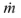= Mass flow rate

The computation compressor efficiency or power involves two steps

1. Determination of the ideal or isentropic (reversible and adiabatic) enthalpy change (h2Isen-h1) of the compression process.
2. Determination of the actual enthalpy change (h2-h1).

The step-by-step calculation based on an EOS:

a. Assume steady state, i.e.

b. Assume the feed composition remain unchanged

c. Calculate suction enthalpy h1=f(P1, T1, and zi) and entropy s1=f(P1, T1, and zi) by EOS

d. Assume isentropic process and set s2Isen = f (P2, T2Isen, zi) = s1 = f (P1, T1, zi).

e. Calculate the ideal enthalpy (h2Isen) at discharge condition for known zi, T2 (or P2) and s2Isen.

f. Calculate the actual enthalpy (h2) at discharge condition for known zi, T2 and P2.

g. Calculate isentropic efficiency by Equation 1: µIsen = (h2Isen – h1)/(h2 – h1)

h. Calculate power by Equation 2: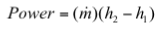Estimating Efficiency – Shortcut Method

The isentropic path exponent (k) or ideal gas heat capacity ratio (k=CP/CV) can be calculated by the correlation presented in the May 2013 TOTM:Where:

T = Temperature, K (°R)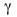= Gas relative density; ratio of gas molecular weight to air molecular weight

A = 0.000272 (0.000151)

The actual discharge temperature based on an isentropic path can be estimated bySolving for the isentropic efficiency,Similarly, the actual discharge temperature based on a polytropic path can be estimated by:Solving the above equation for the polytropic path coefficient (n):Knowing the isentropic path coefficient (k) and the polytropic coefficient (n), one can calculate the polytropic efficiency (ηPoly) by:The isentropic head is calculated by:Similarly, the polytropic head is calculated by:For an isentropic (reversible and adiabatic) process the power is calculated by:Or for a polytropic process the power is calculated by:Alternatively:Where:

Head = Compressor head, m (ft)

Power = Compressor power, kW (HP)

R = Universal gas constant, 848 kg-m/(kmol-K) or (1545 ft-lbf/(lbmol-°R))

PS = Standard condition pressure, kPa (psia)

P1 = Suction pressure, kPa (psia)

P2 = Discharge pressure, kPa (psia)

TS = Standard condition temperature, K (°R)

T1 = Suction temperature, K (°R)

T2 = Discharge temperature, K (°R)

qS = Gas volumetric rate at the standard condition, Sm3/d (scf/day)

Za = Average gas compressibility factor = (Z1+Z2)/2

Z1 = Gas compressibility factor at the suction condition

Z2 = Gas compressibility factor at the discharge condition

MW = Gas molecular weight

The power calculation should be made per stage of compression and then summed for all stages connected to a single driver.

The step-by-step calculation for shortcut method

a. Calculate the isentropic exponent (k) by Equation 3 using the average temperature defined by T = (T1+3T2)/4. This form of average temperature was defined to obtain better match between the rigorous and shortcut method results.

b. Calculate the isentropic efficiency (ηIsen) by Equation 5.

c. Calculate the polytropic coefficient (n) by Equation 7.

d. Calculate the polytropic efficiency (ηPoly) by Equation 8.

e. Calculate the isentropic and polytropic heads by Equations 9 and 10, respectively.

f. Calculate the required power per stage by either Equation 11 or 12.

Case Study

A natural gas mixture is compressed using a three-stage centrifugal compressor. The process flow diagram is shown in Figure 1. For each stage, the measured pressure, and temperature are presented in Table 1. The measured feed composition, flowrates, and calculated molecular weight and relative density are presented in Table 2.Table 1. Measured temperature and pressure for the three stages of compression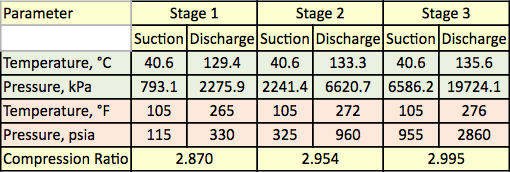Table 2. Gas analysis and flow rate for the three stages of compression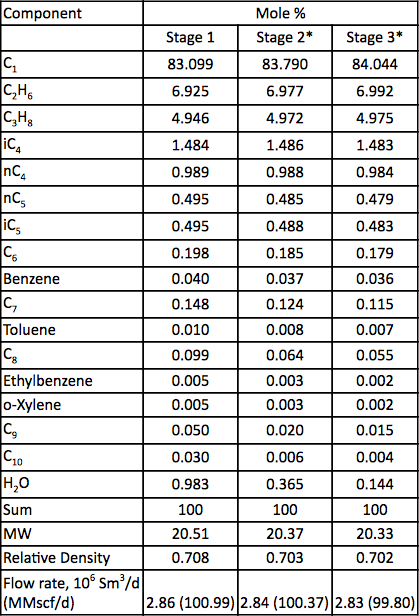* Calculated

Results and Discussions

The process flow diagram shown in Figure 1 was simulated by ProMax software  to perform the rigorous calculations using the SRK EOS. The program calculated polytropic and isentropic efficiencies, heads, and compression power. The program also calculated the isentropic path exponent (k), and polytropic path exponent (n). These calculated results are presented in Table 2 for all three stages under SRK headingings. The calculations performed by ProMax are very similar to the step-by-step of a through h described in the rigorous section. Table 2 also presents the shortcut caculation results for the corresponding values under the shortcut heading. The shortcut calculations are based on the step-by-step of a through f described in the shortcut method section. The error percent between the rigrous method and the shortcut methods for each stage are presented in Table 2, too. Table 2 indicates that excellent agreements are obtained for stages 1 and 2. However, larger deviations are obseved for the isetropic and polytropic exponents of stage 3 due to high pressure operation which deviated too far from ideal gas state conditions.

Table 3. Summary of the rigorous and shortcut calculated results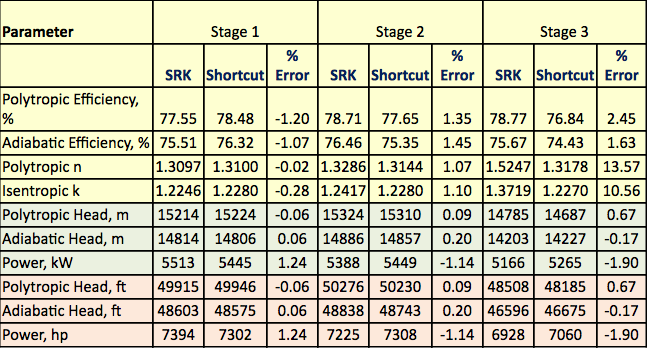Conclusions

Table 2 indicates that there are good agreements between the shortcut and the rigorous results. The differences between the rigorous and shortcut method results for facilities calculations and planning purposes are negligible. For stage 3, due to high-pressure operation and deviating too far from the ideal gas state condition, a larger error is observed for the isentropic exponent (k).

The calculated isentropic exponent (k) in the ProMax  is not the ideal gas state heat capacity (CP/CV) ratio. It is the value of the isentropic exponent that is required to yield an isentropic path from inlet to outlet. Its value is calculated as an integration of that path. Thus it is somewhat of an “average” value representing the true isentropic path. For ideal gases, the value would be equal (CP/CV) ratio.

This error in ‘k’ also illustrates the importance of specifying which correlation is to be used when ordering a performance test (ie, refer to ASME PTC-10 for additional details), so that client and vendor are on the same agreement moving forwards with regard to molecular weight (MW) and k for the test fluid. For further detail refer to reference  and August and September 2010 TOTMs [7, 8].

It may also be worth noting that when trending ‘n’ and the polytropic efficiency to evaluate machine condition, the relative accuracy of measurement instrument/equipment (temperature and pressure transducers) and mapping of compressor performance to the original performance curve (actual gas volume flow rate vs speed), introduces many potential erroneous sources into this daily evaluation.

Note that the accuracy of the shortcut methods is dependent on the values of k and n. The definition of average temperature in the shortcut method was adjusted to obtain a better match between the isentropic path exponent (k) calculated by rigorous method.

To learn more about similar cases and how to minimize operational problems, we suggest attending our G4 (Gas Conditioning and Processing), PF4 (Oil Production and Processing Facilities), ME46 (Compressor Systems–Mechanical Design and Specifications) and ME44 (Fundamentals of Pump and Compressors Systems), courses.

PetroSkills offers consulting expertise on this subject and many others. For more information about these services, visit our website at http://petroskills.com/consulting, or email us at consulting@PetroSkills.com.

Dr. Mahmood Moshfeghian

Reference:

1. Maddox, R. N. and L. L. Lilly, “Gas conditioning and processing, Volume 3: Advanced Techniques and Applications,” John M. Campbell and Company, 2nd Ed., Norman, Oklahoma, USA, 1990.

2. Campbell, J.M., Gas Conditioning and Processing, Volume 2: The Equipment Modules, 9th Edition, 2nd Printing, Editors Hubbard, R. and Snow–McGregor, K., Campbell Petroleum Series, Norman, Oklahoma, 2014.

3. Soave, G., Chem. Eng. Sci., Vol. 27, pp. 1197-1203, 1972.

4. Peng, D. Y., and Robinson, D. B., Ind. Eng. Chem. Fundam., Vol. 15, p. 59, 1976.

5. ProMax 3.2, Bryan Research and Engineering, Inc, Bryan, Texas, 2014.

6. ASME PTC-10, “Performance test Code on Compressors and Exhausters”, 1997.

7. Honeywell, J. “Important Aspects of Centrifugal Compressor Testing-Part 1”, Tip of the Month, August 2010

8. Honeywell, J. “Important Aspects of Centrifugal Compressor Testing-Part 2”, Tip of the Month, September 2010